﻿ Python赋值逻辑的实现 - 自由资讯

# Python赋值逻辑的实现

##### 目录
• 摘要：
• 第一章 引例
• 第二章 Python 的“反直觉”
• 第三章 回答第一章的问题

## 第一章 引例

```# 代码 1

>>> a = 3
>>> b = a
>>> b = 5
>>> a
3```

```# 代码 2

>>> a = [1, 2, 3]
>>> b = a
>>> b = 1024
>>> a
[1024, 2, 3]```

## 第二章 Python 的“反直觉”

```# 代码 3

b = 3
b = b + 5```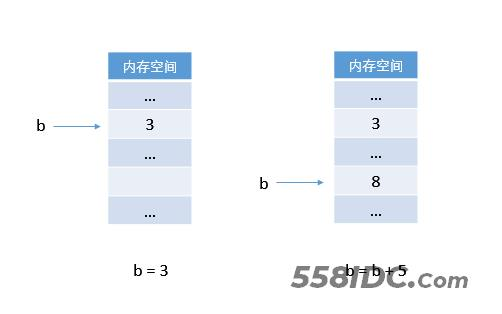`b ← 3b ← b + 5`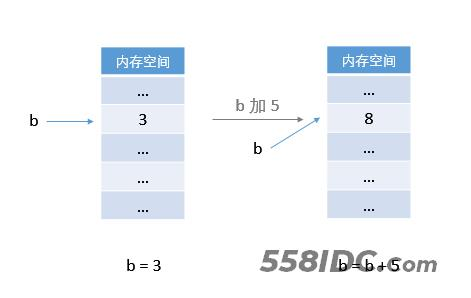```b → 3
b → b + 5```

C 程序更新的是内存单元中存放的值，而 Python 更新的是变量的指向。
C 程序中变量保存了一个值，而 Python 中的变量指向一个值。

Python 的这种操作让数据成为主体，数据与数据之间直接进行交互。

Python 把一切数据都看成「对象」。它为每一个对象分配一个内存空间。 一个对象被创建后，它的 id 就不再发生变化。

id 是 identity 的缩写。意为“身份；标识”。

C 程序为每个变量都分配一个了固定的内存地址，这保证了 C 变量之间的独立性。

C 语言是变量（也即内存地址）之间的交互，Python 是对象（数据）之间的交互。这是两种不同的交互方式。

Python 的赋值更改的是变量的指向关系，因此，对于 Python，从前向后阅读一个赋值表达式会更加容易理解。

```// C 语言
b ← b + 5	// 把 b+5 的值赋给 b```
```# Python
b → b + 5	# 令 b 指向 b + 5```

## 第三章 回答第一章的问题

```# 代码 1

>>> a = 3
>>> b = a
>>> b = 5
>>> a
3```

Python 中所有的数据都是对象，数字类型也不例外。`3` 是一个 `int` 类型的对象，`5` 也是一个 `int` 型的对象。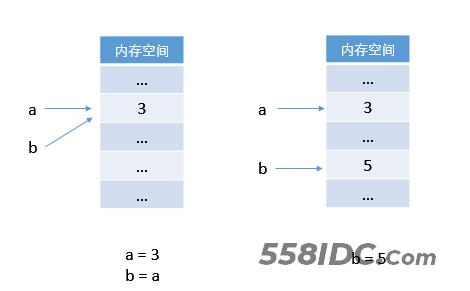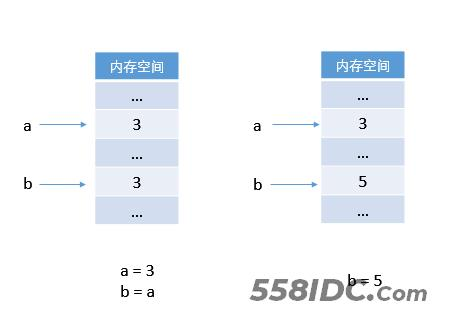Python 中 `b` 首先指向了对象 `3`，然而因为对象之间的独立性，一个对象不能去覆盖另一个对象，则令 `b` 指向对象 `5`，而不是将对象 `3` 在内存中替换为对象 `5`

```# 代码 2

>>> a = [1, 2, 3]
>>> b = a
>>> b = 1024
>>> a
[1024, 2, 3]```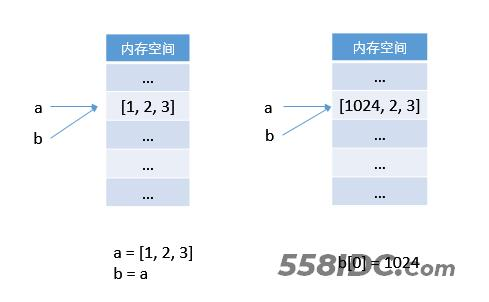```a = [1, 2, 3]
b = a
c = a
d = a
...```

```>>> a = [1, 2, 3]
>>> def change(t):
t = 1024

>>> change(a)
>>> a
[1024, 2, 3]
>>>  ```

```>>> a = 5
n = n + 2

>>> a
5```

• 为什么列表的方法的返回值大多是 `None`
• 为什么字符串的方法的返回值大多是一个新的对象？
• 为什么 Python 中没有自增/自减运算符？
• 为什么有的可变对象传入函数之后，却不能被函数修改“实参”的值？
（比如将上面的 `change` 函数的主体改成 `t = t[1:]`。调用函数之后，`a` 所指向的对象并没有发生改变。）
• ……

1. 数字是一个天然的不可变对象(immutable object)。

2. 为什么 Python 中没有自增(++)、自减(--)运算符？

3. 既然 Python 更改的只是引用关系，那么如何复制一个列表？

```# 答案：
## 1. 使用 list 的 copy 方法
b = a.copy()
## 2. 使用 slice 操作
b = a[:]	# slice 操作返回一个新的对象```
```# 答案：
## 1. 使用 list 的 copy 方法
b = a.copy()
## 2. 使用 slice 操作
b = a[:]	# slice 操作返回一个新的对象```

﻿